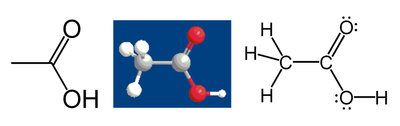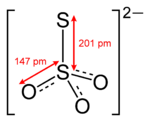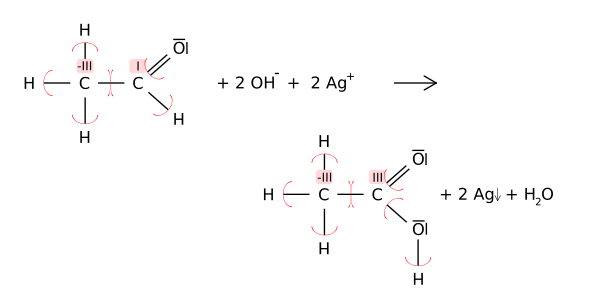# Oxidation state

﻿
Oxidation state

In chemistry, the oxidation state is an indicator of the degree of oxidation of an atom in a chemical compound. The formal oxidation state is the hypothetical charge that an atom would have if all bonds to atoms of different elements were 100% ionic. Oxidation states are typically represented by integers, which can be positive, negative, or zero. In some cases, the average oxidation state of an element is a fraction, such as 8/3 for iron in magnetite (Fe3O4). The highest oxidation state is +8 in the tetroxides of xenon, ruthenium and osmium, while the lowest is −4 for some elements in the carbon group.

The increase in oxidation state of an atom through a chemical reaction is known as an oxidation; a decrease in oxidation state is known as a reduction. Such reactions involve the formal transfer of electrons, a net gain in electrons being a reduction and a net loss of electrons being an oxidation. For pure elements, the oxidation state is zero.

The definition of the oxidation state listed by IUPAC is as follows:

Oxidation state: A measure of the degree of oxidation of an atom in a substance. It is defined as the charge an atom might be imagined to have when electrons are counted according to an agreed-upon set of rules: (1) the oxidation state of a free element (uncombined element) is zero; (2) for a simple (monoatomic) ion, the oxidation state is equal to the net charge on the ion; (3) hydrogen has an oxidation state of 1 and oxygen has an oxidation state of -2 when they are present in most compounds. (Exceptions to this are that hydrogen has an oxidation state of -1 in hydrides of active metals, e.g. LiH, and oxygen has an oxidation state of -1 in peroxides, e.g. H2O2); (4) the algebraic sum of oxidation states of all atoms in a neutral molecule must be zero, while in ions the algebraic sum of the oxidation states of the constituent atoms must be equal to the charge on the ion. For example, the oxidation states of sulfur in H2S, S8(elementary sulfur), SO2, SO3, and H2SO4 are, respectively: -2, 0, +4, +6 and +6. The higher the oxidation state of a given atom the greater its degree of oxidation; the lower the oxidation state the greater its degree of reduction.

## Some general rules for determining oxidation states without use of Lewis structures

Here are general rules for simple compounds without structural formulae:

1. Any pure element (even if it forms diatomic molecules like chlorine, Cl2) has an oxidation state (OS) of zero. Examples of this are Cu or O2.
2. For monatomic ions, the OS is the same as the charge of the ion. For example, S2- has an OS of -2, whereas Li+ has an OS of +1.
3. The sum of OSs for all atoms in a molecule or polyatomic ion is equal to the charge of the molecule or ion, so that the OS of one element can be calculated from the OS of the other elements. For example, in SO32- (sulfite ion), the total charge of the ion is -2, and each oxygen is assumed to have its usual oxidation state of -2. The sum of OSs is then OS(S) + 3(-2) = -2, so that OS(S) = +4.
4. Do not confuse the formal charge on an atom with its formal oxidation state, as these may be different, and often are different, in polyatomic ions. For example, the charge on the nitrogen atom in ammonium ion NH4+ is +1, but the formal oxidation state is -3, the same as it is for nitrogen in ammonia. In this case, the charge on the atom changed, but its oxidation state did not.

To sum up: The algebraic sum of oxidation states of all atoms in a neutral molecule must be zero, while in ions the algebraic sum of the oxidation states of the constituent atoms must be equal to the charge on the ion. This fact, combined with the fact that some elements almost always have certain oxidation states (due to their very high electropositivity or electronegativity), allows one to compute the oxidation states for the remaining atoms (such as transition metals) in simple compounds.

The following rules that are used for initially assigning oxidation states for certain elements, in simple compounds:

• Fluorine has an oxidation state of −1 when bonded to any other element, since it has the highest electronegativity of all reactive elements.
• Halogens other than fluorine have an oxidation state of −1 except when they are bonded to oxygen, nitrogen, or another (more electronegative) halogen. For example, the oxidation state of chlorine in chlorine monofluoride (FCl) is +1. However, in chlorine bromide (or bromine chloride) (ClBr) the oxidation state of Cl is -1.
• Hydrogen has an oxidation state of +1 except when bonded to more electropositive elements such as sodium, aluminium, and boron, as in NaH, NaBH4, LiAlH4, where each H has an oxidation state of -1.
• In compounds, Oxygen typically has an oxidation state of −2, though there are exceptions that are listed below. An example is peroxides (e.g. hydrogen peroxide H2O2) when it has an OS of -1.
• Alkali metals have an oxidation state of +1 in virtually all of their compounds (exception, see alkalide).
• Alkaline earth metals have an oxidation state of +2 in virtually all of their compounds.

Example for a complex salt: In Cr(OH)3, oxygen has an oxidation state of −2 (no fluorine or O-O bonds present), and hydrogen has a state of +1 (bonded to oxygen). So, each of the three hydroxide groups has an oxidation state of −2 + 1 = −1. As the compound is neutral, Cr has an oxidation state of +3.

For molecules with inequivalent atoms of the same element, the algebraic sum method gives only an average oxidation state. We will consider below how to find the oxidation state of each atom with the help of a Lewis structure.

## Calculation of formal oxidation states with a Lewis structure

There are two common ways of computing the oxidation state of an atom in a compound. The first one is the simple algebraic sum technique above, using in compounds that do not require a Lewis structure. The second is used for molecules when one has a Lewis structure.

It should be remembered that the oxidation state of an atom does not represent the "real" charge on that atom: This is particularly true of high oxidation states, where the ionization energy required to produce a multiply positive ion are far greater than the energies available in chemical reactions. The assignment of electrons between atoms in calculating an oxidation state is purely a formalism, but is a useful one for the understanding of many chemical reactions.

For more about issues with calculating atomic charges, see partial charge.

### The Lewis structure

When a Lewis structure of a molecule is available, the oxidation states may be assigned by computing the difference between the number of valence electrons that a neutral atom of that element would have and the number of electrons that "belong" to it in the Lewis structure. For purposes of computing oxidation states, electrons in a bond between atoms of different elements belong to the more electronegative atom; electrons in a bond between atoms of the same element are split equally, and electrons in a lone pair belong only to the atom with the lone pair.

For example, consider acetic acid:The methyl group carbon atom has 6 valence electrons from its bonds to the hydrogen atoms because carbon is more electronegative than hydrogen. Also, 1 electron is gained from its bond with the other carbon atom because the electron pair in the C–C bond is split equally, giving a total of 7 electrons. A neutral carbon atom would have 4 valence electrons, because carbon is in group 14 of the periodic table. The difference, 4 – 7 = –3, is the oxidation state of that carbon atom. That is, if it is assumed that all the bonds were 100% ionic (which in fact they are not), the carbon would be described as C3-.

Following the same rules, the carboxylic acid carbon atom has an oxidation state of +3 (it only gets one valence electron from the C–C bond; the oxygen atoms get all the other electrons because oxygen is more electronegative than carbon). The oxygen atoms both have an oxidation state of –2; they get 8 electrons each (4 from the lone pairs and 4 from the bonds), while a neutral oxygen atom would have 6. The hydrogen atoms all have oxidation state +1, because they surrender their electron to the more electronegative atoms to which they are bonded.

### Inequivalent atoms of an elementStructure of the thiosulfate anion

An example of a molecule with inequivalent atoms of the same element is the thiosulfate ion (S2O32−), for which the algebraic sum rule yields the average value +2 for sulfur. However, the use of a Lewis structure and electron counting shows that the two sulfur atoms are different. The central sulfur is assigned only one valence electron from the S-S bond and no valence electrons from the S-O bonds, compared to six valence electrons for a free sulfur atom, so the oxidation state of the central sulfur is +5. The terminal sulfur atom is assigned the other electron from the S-S bond plus three lone pairs for a total of seven valence electrons, so its oxidation state is -1.

### Redox reactions

Oxidation states can be useful for balancing chemical equations for redox reactions, because the changes in the oxidized atoms have to be balanced by the changes in the reduced atoms. For example, in the reaction of acetaldehyde with the Tollens' reagent to acetic acid (shown below), the carbonyl carbon atom changes its oxidation state from +1 to +3 (oxidation). This oxidation is balanced by reducing two equivalents of silver from Ag+ to Ago.## Elements with multiple oxidation states

Most elements have more than one possible oxidation state. Carbon has nine integer oxidation states, and there are also molecules in which the average degree of oxidation of several carbons is fractional.

#### Integer oxidation states of carbon with examples

1. –4: CH4
2. –3: C2H6
3. –2: CH3Cl
4. –1: C2H2
5. 0: CH2Cl2
6. +1: CHCl2CHCl2
7. +2: CHCl3
8. +3: C2Cl6
9. +4: CCl4

#### Examples of fractional oxidation states for carbon

1. –(6/5): C5H5
2. –(6/7): C7H7+
3. –(10/8): C8H82−

#### Oxygen has 8 different oxidation states

1. –2 in oxides, e.g. ZnO, CO2, H2O
2. –1 in peroxides, e.g. H2O2
3. –(1/2) in superoxides, e.g. KO2
4. –(1/3) in inorganic ozonides, e.g. dioxygenyl, e.g. dioxygenyl hexafluoroarsenate O2+ [AsF6]
5. +1 in O2F2
6. +2 in OF2

Note that, since fluorine is more electronegative than oxygen, O2F2 and OF2 are considered fluorides, rather than a peroxide and an oxide.

### Fractional oxidation states

Fractional oxidation states are often used to represent the average oxidation states of several atoms in a structure. For example, in KO2, the superoxide ion has a charge of -1 divided among two equivalent atoms, so each oxygen is assigned an oxidation state of −½, This ion is described as a resonance hybrid of two Lewis structures, and each oxygen has oxidation state 0 in one structure and -1 in the other.

For the cyclopentadienyl ion C5H5, the oxidation state of C is (-1) + (-1/5) = -6/5. The -1 occurs because each C is bonded to one less electronegative H, and the -1/5 because the total ionic charge is divided among five equivalent C.

If the average refers to atoms that are not equivalent, the average oxidation state may not be representative of each of the atoms. This is true in magnetite Fe3O4, whose formula leads to an average oxidation state of +8/3. In fact, two-thirds of the iron ions are Fe3+, and one-third Fe2+.

Likewise, the ozonide ion O3 has an average oxidation state of -1/3. This ion is V-shaped with a central oxygen, which is not equivalent to the two others and cannot be assumed to have the same oxidation state.

## Oxidation number

The terms oxidation state and oxidation number are often used interchangeably. However, oxidation number is used in coordination chemistry with a slightly different meaning. In coordination chemistry, the rules used for counting electrons are different: Every electron in a metal-ligand bond belongs to the ligand, regardless of electronegativity. Also, oxidation numbers are conventionally represented with Roman numerals, while oxidation states use Indo-Arabic numerals.

## History

The concept of oxidation state in its current meaning was introduced by W. M. Latimer in 1938. Oxidation itself was first studied by Antoine Lavoisier, who held the belief that oxidation was the results of reactions of the elements with oxygen and that the common bond in any salt was based on oxygen.

## Unusual formal oxidation states

Unusual formal oxidation states of metals are important in biochemical processes, the notable ones being Fe(IV) and Fe(V) in Cytochrome P450-containing systems.

Wikimedia Foundation. 2010.

### Look at other dictionaries:

• oxidation state — noun the degree of oxidation of an atom or ion or molecule; for simple atoms or ions the oxidation number is equal to the ionic charge the oxidation number of hydrogen is +1 and of oxygen is 2 • Syn: ↑oxidation number • Hypernyms: ↑number * * *… …   Useful english dictionary

• oxidation state — noun Date: 1942 a positive or negative number that represents the effective charge of an atom or element and that indicates the extent or possibility of its oxidation < the usual oxidation state of sodium is +1 and of oxygen 2 > called also… …   New Collegiate Dictionary

• oxidation state — Chem. the state of an element or ion in a compound with regard to the electrons gained or lost by the element or ion in the reaction that formed the compound, expressed as a positive or negative number indicating the ionic charge of the element… …   Universalium

• oxidation state — noun the state of an atom having a particular oxidation number …   Wiktionary

• oxidation state — see under number …   Medical dictionary

• oxidation–reduction reaction — ▪ chemical reaction Introduction also called  redox reaction        any chemical reaction in which the oxidation number of a participating chemical species changes. The term covers a large and diverse body of processes. Many oxidation– reduction… …   Universalium

• Oxidation number — Not to be confused with oxidation state. Oxidation numbers of carbon and hydrogen in ethane In coordination chemistry, the oxidation number of a central atom in a coordination compound is the charge that it would have if all the ligands were… …   Wikipedia

• oxidation number — ▪ chemistry also called  Oxidation State,        the total number of electrons (electron) that an atom either gains or loses in order to form a chemical bond (chemical bonding) with another atom.       Each atom that participates in an oxidation… …   Universalium

• Oxidation of primary alcohols to carboxylic acids — The oxidation of primary alcohols to carboxylic acids is an important oxidation reaction in organic chemistry. When a primary alcohol is converted to a carboxylic acid, the terminal carbon atom increases its oxidation state by four. Oxidants able …   Wikipedia

• oxidation number — a number assigned to each atom in a molecule or ion that represents the number of electrons theoretically gained (positive oxidation numbers) or lost (negative numbers) in converting the atom to the elemental form. Oxidation numbers are assigned… …   Medical dictionary